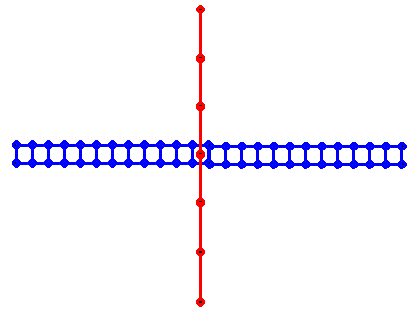# A Dipole with a Boom Simulating Thick Wire

Simulating a conductive Boom near a Dipole element.

A possible method of dealing with the thick vs. thin situation is to model with real wires with a network of smaller model wires that are connected to each other. This was mentioned on the page dealing with explaining the limitations of the thin wire model.

We start by pointing out that a rod (or thick wire) can be simulated by a network of much smaller wires. In the example below, a wire segment is represented by a triangular lattice of smaller wires.It is not necessary to use a triangular grid. A grid with any polygon as the circumferance might work. However it is also possible in many cases to be even simpler just to get the general effect. In the case of the near field of the dipole we can try just a rectangular grid as shown below.This is simpler and has a smaller number of wire grid elements than the triangular gridding. Once the rectangular segments are stacked end-to-end, the wire structure will resemble a ladder. Since the near field of the dipole is dominant parallel to the dipole, the "ladder" cross-bars are also turned parallel to the dipole.

Below is a figure of a dipole (thin rod shown vertical) that is near a conductive boom (thicker rod shown horizontal). To simulate the horizontal boom, it will be made into the "ladder" structure.The figure below shows conceptually the dipole and the boom after they have been segmented for analysis. The dipole, represented in the color red, is modeled as a single thin-wire structure. The boom, represented in blue, is modeled in the "ladder" structure of cross-connected parallel wire segments.The above picture is conceptual and not to scale. In a real model, it is necessary to keep all of the wire segments nearly the same size. The limit in segment size variation (found in the User's Manual and the quick reference) is [longest segment]/[shortest segment] < 100

Also when wires lay close together, the wire diameter set into the model must be much, much smaller than the closest spacing. For best results, the length of the wire segments making up the "ladder" boom section shouldn't be any longer than the smallest spacing between the wires in parallel. To meet all of these requirements requires a large number of segments even for a small problem.

In the problem being considered, the dipole is described with 51 points joined to make 50 segments. The dipole is fed at the center point. The dipole is 0.5 meters long; therefore each segment is 1 cm long. The boom is composed of two parallel wires each 0.5 meters long and are also divided into 1 cm segments. These wires are at right angles to the dipole wire element and spaced 4 cm away. Therefore, the dipole is insulated from the boom for this problem. The two wires making up the boom are 2 cm apart and are joined together like a ladder by cross connections running between the description points. All of the wires making up the model are 0.001 meter i.e. 1 mm in radius.

The full input file can bee obtained here: DBOOM.DAT (9K) (This file also contains a single case of a single wire as the boom to show that a simple single wire has no effect.)

The dipole by itself and the dipole with the ladder boom simulation were run for multiple frequency points to gauge the effect on feedpoint impedance of the dipole. The plots below show the input resistance vs. frequency and the reactance vs. frequency on separate plots.A half-wave dipole in free space resonates at a frequency that is lower than the free-space half-wavelength. Therefore a 0.5 meter dipole will be above the resonance frequency at 300 MHz as is confirmed by the model showing positive reactance number. Even though the dipole is not "at resonance" in this example, the shift in effective response can still be noted from the curves. Note that both the resistance and the reactance curves show a shift of about 400 kHz. To get the same impedance, it is necessary to go to a higher frequency with the boom than it is without. In one sense, this means that the dipole appears to be effectively shorter with the boom than it would be in free space. The dipole would have to be lengthened slightly in order for it to act "the same" as it would in free space. This effective change in frequency is very important when making yagi arrays. The element lengths needed for maximum gain at a particular frequency will be different with a conductive boom than calculated from free space relations due to the exact phasing needed between the elements.

The dipole gain and pattern is not much affected by the boom however. About the only thing effected in the case of a single dipole is the impedance. There is a small effect on linear gain in some directions that is less than one part in a thousand. This is due to the small amount of radiation from the cross-wise currents on the boom. The boom is not a very effective radiator in that direction because it is only 2 cm wide.

or else go to next page to consider the effects on a yagi antenna.

Or

Including:

Goto Full User's Manual (from Thesis)